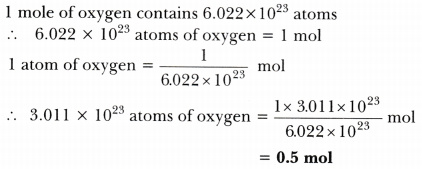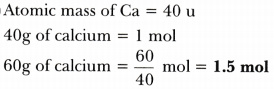# Calculate the number of moles present in: 3.011 X 10^23 number of oxygen atoms. 60 g of calcium

Calculate the number of moles present in:

1. 3.011 X 10^23 number of oxygen atoms.
2. 60 g of calcium

[Given that atomic mass of Ca = 40 u, Avogadro No. = 6.022 X 10^23]

1.2.